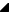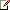﻿ TRSChartAxis Methods# TRSChartAxis MethodsMethods

Name

DescriptionAssign(TPersistent)

Copies the contents of another similar object to the current objectNotes to Inheritors

Most objects override Assign to handle the assignment of properties from similar objects. When overriding Assign, call the inherited method if the destination object cannot handle the assignment of properties from the class of the Source parameterAxisToPixel(TRSChartValueType)

Overloaded.  Converts from the chart value parameter to a pixel coordinate of the Owner RSChartPanel.TRSCustomChartPanel component. Based on the     ZoomMinimum and     ZoomMaximum range of the chart axis, this routine converts the parameter value into a pixel coordinate. Unlike the overloaded AxisToPixel method, this method assumes that the visual pixel range of the chart axis is defined by the chart panel's     ChartRect property. The chart axis uses the bounds of the rectangle that apply to its location, e.g., a BottomAxis chart axis would use the ChartRect's Left=ZoomMinimum and Right=ZoomMaximum fields for the calculation (the rectangle is also adjusted if StartPosition and EndPosition properties are not their defaults).

Use one of the AxisToPixel methods anytime you need to convert from internal chart axis coordinates to a visual pixel coordinate. For example, if the chart axis has a zoomed range from 0 to 100 and the ChartRect is Left=150 and Right=500, this function would return:
AxisToPixel( 0 ) = 150
AxisToPixel( 50 ) = 325
AxisToPixel( 100 ) = 500

Use one of the     PixelToAxis methods to convert back from pixel coordinates to chart coordinates.AxisToPixel(TRSChartValueType,TCanvasRect)

Overloaded.  Converts from the chart value parameter to a pixel coordinate of the specified ChartRect parameter. Based on the     ZoomMinimum and     ZoomMaximum range of the chart axis, this routine converts the parameter value into a pixel coordinate. Unlike the overloaded AxisToPixel method, this method assumes that the visual pixel range of the chart axis is defined by the ChartRect property. The chart axis uses the bounds of the rectangle that apply to its location, e.g., a BottomAxis chart axis would use the ChartRect's Left=ZoomMinimum and Right=ZoomMaximum fields for the calculation (the rectangle is also adjusted if StartPosition and EndPosition properties are not their defaults).

Use one of the AxisToPixel methods anytime you need to convert from internal chart axis coordinates to a visual pixel coordinate. For example, if the chart axis has a zoomed range from 0 to 100 and the ChartRect is Left=150 and Right=500, this function would return:
AxisToPixel( 0 ) = 150
AxisToPixel( 50 ) = 325
AxisToPixel( 100 ) = 500

Use one of the     PixelToAxis methods to convert back from pixel coordinates to chart coordinates.AxisToPixel(TRSLogarithmicType,TRSChartValueType,TCanvasRect,TRSChartValueType,TRSChartValueType,Boolean)CalculateBounds(TCanvas,TCanvasRect)

Sets the chart axis' Width and Height to their proper values based on the ARect parameter.Tip

Usually, this method does not need to be called directly as it is automatically called when the chart axis is painted. However, when the chart axis has not been painted yet (e.g., the OnCreate event of the form) and you want to use the chart axis, call this method first.Changed

Overloaded. Represents method Changed. (Overrides RSChartPanel.TRSChartGraphic.Changed.)Changed(TObject)DrawAxis(TCanvas,TCanvasRect)

Represents method DrawAxis(TCanvas,TCanvasRect).DrawAxisLine(TCanvas,TCanvasRect)

Represents method DrawAxisLine(TCanvas,TCanvasRect).DrawGrid(TCanvas,TCanvasRect)

Represents method DrawGrid(TCanvas,TCanvasRect).DrawLabels(TCanvas,TCanvasRect)

Represents method DrawLabels(TCanvas,TCanvasRect).DrawTitle(TCanvas,TCanvasRect)

Represents method DrawTitle(TCanvas,TCanvasRect).FormatValue(TRSChartValueType)

Returns a string representation of the chart value based on the     Format or     DateTimeFormat properties. Based on the     IsDateTime method, the FormatValue function converts the floating point value to a string using the     Format property or a date time string using the     DateTimeFormat property.

Use the FormatValue method to convert a value to a string using the formatting of the chart axisGetAxisLabelStyle

Represents method GetAxisLabelStyle.GetHalfRect(TCanvasRect,Boolean)

Represents method GetHalfRect(TCanvasRect,Boolean).GetLabelSpacing(TCanvas,TCanvasRect,TRSChartValueType,TRSChartValueType)

Represents method GetLabelSpacing(TCanvas,TCanvasRect,TRSChartValueType,TRSChartValueType).GetLogCycleAxis(TCanvasRect,TRSChartValueType,TRSChartValueType,TRSChartValueType)

Represents method GetLogCycleAxis(TCanvasRect,TRSChartValueType,TRSChartValueType,TRSChartValueType).GetLogCycleZoomChart(TCanvasRect,TRSChartValueType,TRSChartValueType,TRSChartValueType)

Represents method GetLogCycleZoomChart(TCanvasRect,TRSChartValueType,TRSChartValueType,TRSChartValueType).Initialize

Represents method Initialize. (Overrides RSChartPanel.TRSChartGraphicArea.Initialize.)InitializeEvents

Represents method InitializeEvents. (Overrides RSChartPanel.TRSChartGraphicArea.InitializeEvents.)InternalDraw(TCanvas,TCanvasRect)

Represents method InternalDraw(TCanvas,TCanvasRect). (Overrides RSChartPanel.TRSChartGraphicArea.InternalDraw(TCanvas,TCanvasRect).)IsDateTime

Returns whether the axis is displaying chart values using a date time string representation or a floating point string representation. The chart axis displays date time values when the   ActiveChart (the first visible chart in the   Charts list) specifies its   IsDateTime property to True. When the chart axis displays date time values, the   FormatValue method uses the   DateTimeFormat property for formatting. Otherwise, the FormatValue method uses the   Format property for formatting.Pan(Integer)

Overloaded.  Moves the visual window, specified by the     ZoomMinimum and     ZoomMaximum properties, by the amount. The Amount parameter, which is based on pixels, is first converted into the equivalent amount based on chart axis values. Specifying a positive amount moves the zoomed window right and a negative amount moves it left.

Use the overloaded Pan method to move the visual window using chart axis values directly.

The Pan method does nothing if the chart axis is not zoomed in (     Zooming = True).Pan(TRSChartValueType)

Overloaded.  Moves the visual window, specified by the     ZoomMinimum and     ZoomMaximum properties, by the amount. The Amount parameter is based on chart axis values. Specifying a positive amount moves the zoomed window right and a negative amount moves it left.

Use the overloaded Pan method to move the visual window using pixel amounts directly.

The Pan method does nothing if the chart axis is not zoomed in (     Zooming = True).PixelToAxis(TCanvasPixel,Boolean)

Overloaded.  Converts from a canvas pixel coordinate to a chart axis coordinate. Based on the     ZoomMinimum and     ZoomMaximum range of the chart axis, this routine converts the parameter value into a chart axis coordinate. Unlike the overloaded PixelToAxis method, this method assumes that the visual pixel range of the chart axis is defined by the chart panel's ChartRect property (obtained through the Owner property). The chart axis uses the bounds of the rectangle that apply to its location, e.g., a BottomAxis chart axis would use the ChartRect's Left=ZoomMinimum and Right=ZoomMaximum fields for the calculation (the rectangle is also adjusted if StartPosition and EndPosition properties are not their defaults).

Use one of the PixelToAxis methods anytime you need to convert from a canvas pixel coordinate to the internal chart axis coordinates. For example, if the chart axis has a zoomed range from 0 to 100 and the ChartRect is Left=150 and Right=500, this function would return:
PixelToAxis( 150 ) = 0
PixelToAxis( 325 ) = 50
PixelToAxis( 500 ) = 100

Use one of the AxisToPixel methods to convert back from chart coordinates to canvas pixel coordinates.PixelToAxis(TCanvasPixel,TCanvasRect,Boolean)

Overloaded.  Converts from a canvas pixel coordinate to a chart axis coordinate. Based on the     ZoomMinimum and     ZoomMaximum range of the chart axis, this routine converts the parameter value into a chart axis coordinate. This method uses the ChartRect parameter to specify the visual pixel range of the chart axis. The chart axis uses the bounds of the rectangle that apply to its location, e.g., a BottomAxis chart axis would use the ChartRect's Left=ZoomMinimum and Right=ZoomMaximum fields for the calculation (the rectangle is also adjusted if StartPosition and EndPosition properties are not their defaults).

Use one of the PixelToAxis methods anytime you need to convert from a canvas pixel coordinate to the internal chart axis coordinates. For example, if the chart axis has a zoomed range from 0 to 100 and the ChartRect is Left=150 and Right=500, this function would return:
PixelToAxis( 150 ) = 0
PixelToAxis( 325 ) = 50
PixelToAxis( 500 ) = 100

Use one of the AxisToPixel methods to convert back from chart coordinates to canvas pixel coordinates.PixelToAxis(TRSLogarithmicType,TCanvasPixel,TCanvasRect,TRSChartValueType,TRSChartValueType,Boolean)SizeUnzoomed

Returns the size of the axis (Maximum-Minimum)SizeZoomed

Returns the size of the zoomed axis (ZoomMaximum-ZoomMinimum)UnboundPixelToAxis(TCanvasPixel,TCanvasRect)

Represents method UnboundPixelToAxis(TCanvasPixel,TCanvasRect).Unzoom

Unzooms the axis to its full range. The Unzoom method changes the visual portion of the chart axis' range to its full range (e.g.,   ZoomMinimum =   Minimum and   ZoomMaximum =   Maximum).UpdateAxisValues

Represents method UpdateAxisValues.UpdateChartAxisInfo

Represents method UpdateChartAxisInfo.ValueChanged(TRSCustomChart)

Represents method ValueChanged(TRSCustomChart).VisibleChanged(TRSCustomChart)Zoom(TRSChartValueType,Single)

Overloaded.  Zoom a specific part of the axis range by specifying the center (in chart values, not pixel values) and zoom magnification amount. The Zoom method changes the     ZoomMinimum and     ZoomMaximum properties to zoom the axis into that visual portion of the range. It allows you to change the portion of the axis that is being visually displayed.

The overloaded Zoom method zooms the axis using chart values. The ZoomPixelArea method zooms the axis using canvas rectangle values instead of chart values.Zoom(TRSRect)

Overloaded.  Zoom the axis in on (or magnify) a portion of the axis' range. The Zoom method accepts a rectangle using chart axis coordinates and changes the     ZoomMinimum and     ZoomMaximum properties to zoom the axis into that visual portion of the range. It allows you to change the portion of the axis that is being visually displayed.

The ZoomPixelArea method zooms the axis using canvas rectangle values instead of chart values.ZoomAmount

Returns the current zoom amount or magnification. A value of 1 means no magnification.ZoomPixelArea(TCanvasRect)

Overloaded.  Zoom the axis in on (or magnify) a portion of the axis' range. The Zoom method accepts a canvas pixel rectangle and changes the     ZoomMinimum and     ZoomMaximum properties to zoom the axis into that visual portion of the range. It allows you to change the portion of the axis that is being visually displayed.

The overloaded Zoom method zooms the axis using chart values instead of canvas rectangle values.

Top

RiverSoftAVG Charting Component Suite (RCCS) © 2005-2015, Thomas G. Grubb